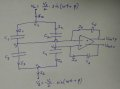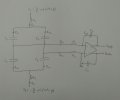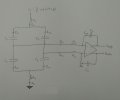# How to solve a circuit with a fully differential amplifier?

#### Lee Yakir

Joined Feb 25, 2017
6
Hello guys, I'm having some trouble with solving the next circuit (was simplified from a more complicated one with extra resistors). I'm a bit rusty with analog devices and would be thankful for some help how to solve that circuit. Hopefully I'd be able to solve the more complicated one by myself after solving the simplified oneMy main issue is how to deal with the amplifier itself (let's assume it's ideal, if it helps solving it) and using the phasors. As I said, a bit rusty..

The circuit includes 2 SINE inputs, and 2 Vout outputs.
Known parameters: capacitors C1 to C6 and the inputs.
Need to find the relation between the total output voltage (VoutP-VoutN) and the parameters above.#### GopherT

Joined Nov 23, 2012
8,009
The extra resistors were there so one can calculate time constants (R*C) and the delays needed for filters. Without resistors, the caps charge and discharge instantly.

Also, the op amps will lock up over time (seconds to minutes) because there is no path to ground for the inputs because there is no current flow through a capacitor - most likely if the op amps are jFet inputs with extraordinarily high I put impedence.

#### Lee Yakir

Joined Feb 25, 2017
6
The extra resistors were there so one can calculate time constants (R*C) and the delays needed for filters. Without resistors, the caps charge and discharge instantly.

Also, the op amps will lock up over time (seconds to minutes) because there is no path to ground for the inputs because there is no current flow through a capacitor - most likely if the op amps are jFet inputs with extraordinarily high I put impedence.
My bad, GopherT. Here is the complete circuit. Does it make more sense now and possible to solve? All capacitors and resistors are given.#### Veracohr

Joined Jan 3, 2011
771
Superposition.

Replace one source with ground, solve for the other. Then switch, and then add the two results.

#### Kjeldgaard

Joined Apr 7, 2016
476
My bad, GopherT. Here is the complete circuit. Does it make more sense now and possible to solve? All capacitors and resistors are given.
View attachment 121386
In the schematic of post #3, there is still no DC path from the amplifier inputs to ground.

•GopherT

#### GopherT

Joined Nov 23, 2012
8,009
My bad, GopherT. Here is the complete circuit. Does it make more sense now and possible to solve? All capacitors and resistors are given.
View attachment 121386
The voltage in each sub circuit that include each input can be virtually any value. Capacitive coupling transfers a signal in response to the RATE OF CHANGE of the voltage and is independent of the actual DC offset voltage.

Think about DC bias of a transistor's base voltage and an AC coupled signal input to that biased point. Your circuit is similar. You need a resistor network to ground in order to calculate the voltage. Voltages that do not connect to ground are called "floating". That untethered (floating) circuit segment has no place in a differential amplifier. There is no answer to your circuit.

#### Lee Yakir

Joined Feb 25, 2017
6
Guys thanks for the support.
If I do superposition, let's assume I have the next circuit.
In that case I do have a resistor network to ground (R3+R4, isn't that?).

So how can I continue solving that "half" circuit? Vu is an AC input.
My main problem/question is how do deal with the amplifier itself? Don't I miss it's amplifying factor somewhere? What are the voltages in its inputs? Can I assume 0 on both amplifier inputs since it's ideal one? If so, what is the voltage difference it's supposed to amplify?
AS you can see, I'm totally in the dark in here#### BR-549

Joined Sep 22, 2013
4,928
Me too. What are you trying to do?

Are you just looking for a math solution?

#### Lee Yakir

Joined Feb 25, 2017
6
Me too. What are you trying to do?

Are you just looking for a math solution?
Yeah, I'm looking for a math solution to describe VoutP and VoutN as functions of the resistors, the capacitors and the input voltages

#### BR-549

Joined Sep 22, 2013
4,928
May I ask where you got the circuit? What is it's function?

#### Lee Yakir

Joined Feb 25, 2017
6
May I ask where you got the circuit? What is it's function?
To amplify the voltage, based on the differences between the capacitors. Basically, if all capacitors are equal and so are the resistors, the gain should be 1.
Any idea how to get the equations?

#### BR-549

Joined Sep 22, 2013
4,928
So......we are going to measure the gain to compare capacitors?